# µMAG Standard Problem #4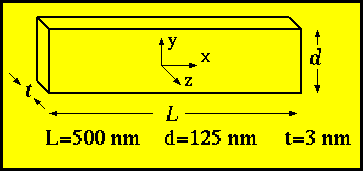Problem brainstormed by Bob McMichael, Roger Koch and Thomas Schrefl; developed by Jason Eicke and Bob McMichael.

Please send comments to rmcmichael@nist.gov and join the µMAG discussion e-mail list for ongoing discussion.

Sets of solutions are available.

## Specifications

Standard problem #4 is focused on dynamic aspects of micromagnetic computations.  The initial state is an equilibrium  s-state  such as is obtained after applying and slowly reducing a saturating field along the [1,1,1] direction to zero.  Fields of magnitude sufficient to reverse the magnetization of the rectangle  are applied to this initial state and the time evolution of the magnetization as the system moves towards equilibrium in the new fields are examined.  The problem will be run for two different applied fields.

#### Geometry:

A film of thickness, t=3 nm, length, L=500 nm and width, d=125 nm will be used.

#### Material parameters:

Similar to  Permalloy:
A = 1.3e-11 J/m (1.3e-6 erg/cm)
Ms = 8.0e5 A/m (800 emu/cc)
K = 0.0
The dynamics, calculated either using the Landau-Lifshitz equation,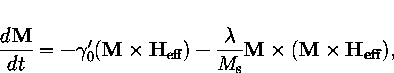or the Gilbert equation,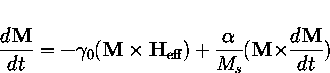will use parameters,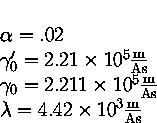Note that these parameters are related by the equations: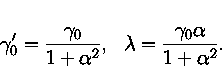Applied Fields:

Two switching events will be calculated using fields applied in the x-y plane of different  magnitude and direction.

• Field 1:  µ0Hx=-24.6 mT, µ0Hy= 4.3 mT, µ0Hz= 0.0 mT  which is a field approximately 25 mT, directed 170 degrees counterclockwise from the positive x axis
• Field 2:  µ0Hx=-35.5 mT, µ0Hy=-6.3 mT, µ0Hz= 0.0 mT which is a field approximately 36 mT, directed 190 degrees counterclockwise from the positive x axis
Each field will be applied instantaneously at t=0 to the equilibrium s-state .

Comparison:

Two outputs are desired for comparison:

• The (x,y,z) components of the spatially averaged magnetization of the sample as a function of time from t=0 until the sample reaches equilibrium in the new field
• An image of the  magnetization at the time when the x-component of the spatially averaged magnetization first crosses zero
The magnetization values in the time series data should be normalized by Ms.  The time series data is desired so that a detailed comparison can be made between solutions.   The magnetization images are to check for any differences in the  reversal mechanisms  if the time data between solutions is different.

Please see the µMAG standard problem strategy page for information on publicizing your results.